# Module 3 'Reactive Chemistry' Practice Test | Year 11 Chemistry

Test your exam-readiness for Year 11 Chemistry Module 3 'Reactive Chemistry' with 10 must-know exam-style questions with solutions.

## Practice test for Year 11 Chemistry Module 3 ‘Reactive Chemistry’

The most effective way to prepare for a chemistry exam is to practise as many exam-style questions as possible. That’s why we have put together a Module 3 Reactive Chemistry Practice Test consisting of 10 challenging exam-style questions. Completing this practice test will give you a competitive edge for your next exam.

This module 3 ‘Reactive Chemistry’ practice test covers topics on:

## Module 3 ‘Reactive Chemistry’ Practice Test

### Instructions

General instructions for sitting the Module 3 Chemistry Practice Test are outlined below.

• Reading time is 5 minutes
• Working time is 45 minutes
• Total marks is 30
• Attempt all questions.
• You must write in black pen.
• Do not write in pencil.
• You may only use a pencil for drawing diagrams and graphs.
• All necessary working should be shown in every question.
• Marks may be deducted for careless or badly arranged work.
• Set out all working for numerical questions and provide appropriate units.
• You may refer to a formulae sheet and data sheet.
• Calculators approved by NESA may be used.

In the final HSC Chemistry exam, you will have approximately 1.8 minutes per mark.

In practice exams, you should aim to answer questions at a pace of 1.5 minutes per mark.

To simulate real exam conditions:

• Close all unnecessary tabs and turn off notifications on your device.
• Set up a timer for 45 minutes.
• Complete this exam in a quiet environment where you will not be disturbed for the duration of the exam.

Once you have completed the Module 3 ‘Reactive Chemistry’ practice test, mark each question carefully using the provided solutions and note any areas for improvement. Remember that marking practice exams is just as important as answering the questions.

### Chemistry formulas

 $n=\dfrac{m}{MM}$ $c=\dfrac{n}{v}$ $PV=nRT$

 Volume of 1 mole of ideal gas: at 100 $\small \text{kPa}$ and at 0$\small \degree \text{C}$ (273.15 $\small \text{K}$) 22.71 $\small \text{L}$ at 25$\small \degree \text{C}$ (298.15 $\small \text{K}$) 24.79 $\small \text{L}$ Gas constant 8.314 $\small \text{J mol}^{-1}\text{K}^{-1}$

### Question 1: Ionic equations

Write balanced full formula, full ionic and net ionic forms of the following equation:

 a) silver nitrate(aq) + barium chloride(aq) → silver chloride(s) + barium nitrate(aq) 3 b) aluminium metal + nitric acid → aluminium nitrate + hydrogen gas 3

See Question 1 Solution

### Question 2: Metal activity series

A student performed three tests to investigate the relative activity of metals. In each test, a metal strip was placed in a solution containing ions of a different metal. The results from this experiment are shown in the diagrams below: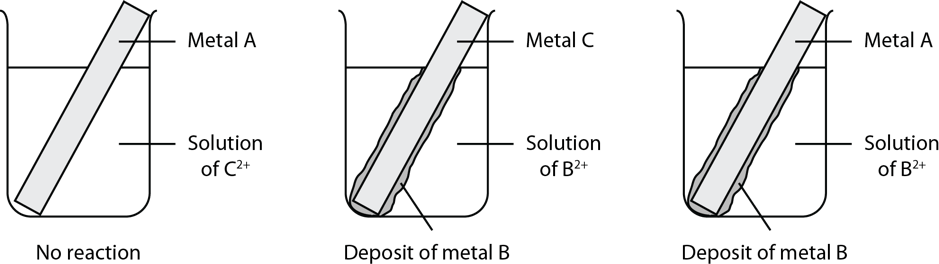What is the order of activity of the metals, based on these results?

 (A) A > C > B (B) B > A > C (C) C > B > A (D) C > A > B

See Question 2 Solution

### Question 3: Oxidation states

Which of the following shows the greatest change in the oxidation state of the metal atom?

 (A) $\small Mn(OH)_3$ to $\small {MnO_4}^-$ (B) $\small V_2O_5$ to $\small VO^{2+}$ (C) $\small MnO_2$ to $\small Mn^{2+}$ (D) $\small {Cr_2O_7}^{2-}$ to $\small Cr_2O_3$

See Question 3 Solution

### Question 4: Oxidising and reducing agents

Identify the oxidising agent for the following reaction:

${5CO}_{(g)} + {I_2O_5}_{(s)} \rarr {5CO}_{2(g)} + I_{2(s)}$

 (A) $\small {CO}$ (B) $\small {C^{2+}}$ (C) $\small {I_2O_5}$ (D) $\small {I^{5+}}$

See Question 4 Solution

### Question 5: Redox reactions

An iron nail was placed in a beaker containing 100 $\small \text{mL}$ of 0.120 $\small \text{mol L}^{-1}$ copper(II) sulfate solution.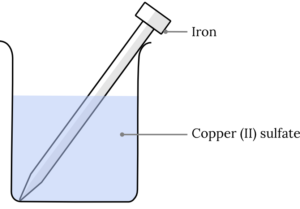After several hours, the dark blue colour of the solution had become lighter and a red-brown deposit had formed on the piece of iron metal.

 a) Write the full formula equation for the redox reaction occurring if iron(II) ions are present in the solution. 1 b) Write the half-equations for the above reaction and label them as oxidation or reduction. 2

See Question 5 Solution

### Question 6: Galvanic cells

An experiment was set up as shown.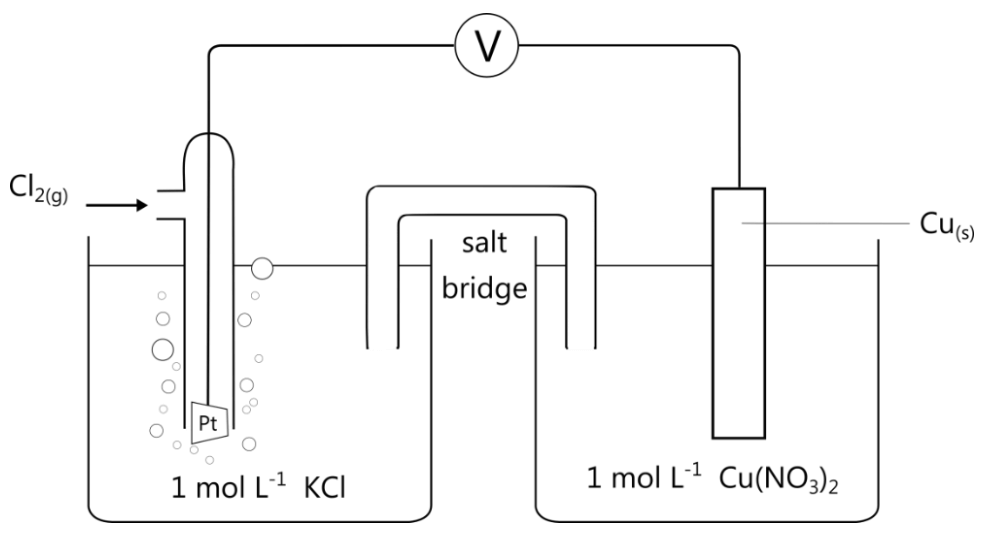Which of the following statements is correct?

 (A) The chlorine gas is the anode. (B) The copper electrode is the anode. (C) The platinum electrode is the anode. (D) There is no anode because chlorine is a gas.

See Question 6 Solution

### Question 7: Cell potential

The diagram below shows a galvanic cell.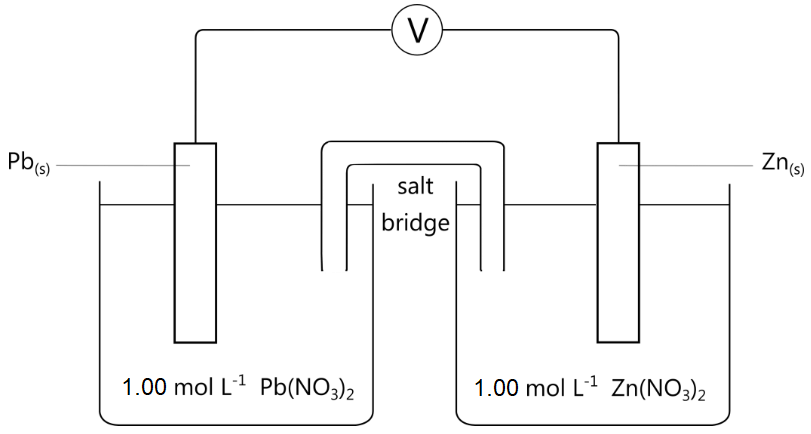a) Write half-equations and the overall equation for the reaction occurring above. 2 b) Calculate the standard potential of the cell. 1 c) The lead electrode gained 0.752 $\small \text{g}$ in mass throughout the course of a reaction. If the zinc half-cell initially contained 150 $\small \text{mL}$ of 1.00 $\small \text{M}$ zinc nitrate solution, what is the final concentration of zinc ions? 3

See Question 7 Solution

### Question 8: Predicting spontaneity using cell potentials

Which of the following redox reactions can NOT be used to produce electricity in an electrochemical cell?

 (A) ${2Ag^+}_{(s)} + {Ni}_{(s)} \rarr {2Ag}_{(s)} + {Ni^{2+}}_{(aq)}$ (B) ${Ca^{2+}}_{(aq)} + {Zn}_{(s)} \rarr {Ca}_{(s)} + {Zn^{2+}}_{(aq)}$ (C) ${Cu^{2+}}_{(aq)} + {Zn}_{(s)} \rarr {Cu}_{(s)} + {Zn^{2+}}_{(aq)}$ (D) ${3Ni^{2+}}_{(aq)} + {2Al}_{(s)} \rarr {3Ni_{(s)}} + {2Al^{3+}}_{(aq)}$

See Question 8 Solution

### Question 9: Rates of reaction

Calcium carbonate reacts with nitric acid to produce water, carbon dioxide gas, and a salt.

 a) Write a balanced chemical equation to represent this reaction. 1 b) Outline two methods that can be used to increase the rate of reaction of calcium carbonate in nitric acid. Justify your answer with reference to the collision theory. 5

See Question 9 Solution

### Question 10: Collision theory

The graphs shown below illustrate the relationship between the number of collisions and kinetic energy at three different temperatures in a chemical reaction.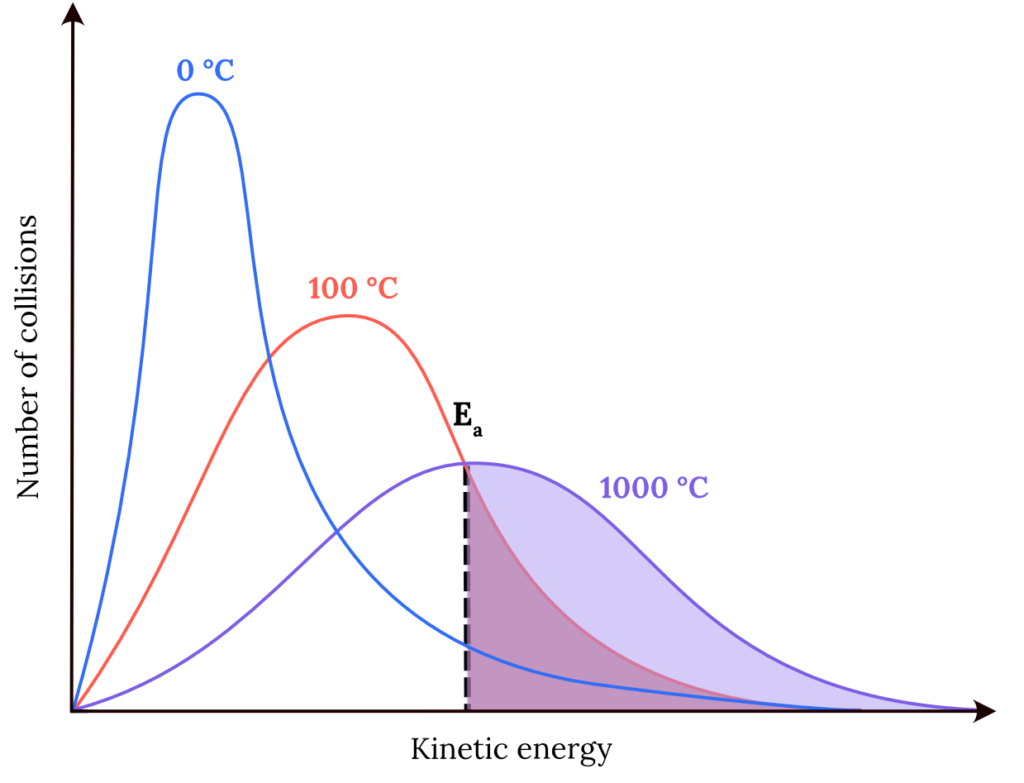Explain, in terms of collision theory, what causes reaction rates to increase with increasing temperature. (4 marks)

See Question 10 Solution

## Solutions for Module 3 Reactive Chemistry Practice Test

 Q Answer 1 Part a)Full formula equation: ${2AgNO_3}_{(aq)} + {BaCl_2}_{(aq)} \rarr {2AgCl}_{(s)} + {Ba(NO_3)_2}_{(aq)}$ Full ionic equation: $\small {2Ag^+}_{(aq)} + {2NO_3^-}_{(aq)} + {Ba^{2+}}_{(aq)} + {2Cl^-}_{(aq)} \rarr {Ba^{2+}}_{(aq)} + {2AgCl}_{(s)}+ {2NO^{3-}}_{(aq)}$ Net ionic equation: ${Ag^+}_{(aq)} + {Cl^-}_{(aq)} \rarr {AgCl}_{(s)}$ Part b)Full formula equation: ${2Al}_{(s)} + {6HNO_3}_{(aq)} → {2Al(NO_3)_3}_{(aq)} + {3H_2}_{(g)}$ Full ionic equation: ${2Al}_{(s)} + {6H^+}_{(aq)} + {6NO_3^-}_{(aq)} \rarr {2Al^{3+}}_{(aq)} + {6NO_3^-}_{(aq)} + {3H_2}_{(g)}$ Net ionic equation: ${2Al}_{(s)} + {6H^+}_{(aq)} \rarr {2Al^{3+}}_{(aq)} + {3H_2}_{(g)}$ Back to Question 1 2 Answer (D) More active metals displace less active metals from solution.Metal A didn’t displace Metal C in solution. Hence, Metal A is less reactive than Metal C (A < C).Metal C displaced Metal B in solution. Hence, Metal C is more active than Metal B (C > B).Metal A displaced Metal B in solution. Hence, Metal A is more active than Metal B (A > B).Therefore, based on these results, the order of activity of the metals is C > A > B.Back to Question 2 3 Answer (A)For $\small {\overset{\textcolor{ff5748}{3+}}{Mn}}{(OH)_3}$ to $\small {\overset{\textcolor{ff5748}{7+}}{Mn}}{O_4}^-$, the change in the oxidation state for $\small Mn$ is -4.For $\small {\overset{\textcolor{ff5748}{5+}}{V_2}}{O_5}$ to $\small {\overset{\textcolor{ff5748}{4+}}{V}}O^{2+}$, the change in the oxidation state for $\small V$ is -1.For $\small {\overset{\textcolor{ff5748}{4+}}{Mn}}O_2$ to $\small {\overset{\textcolor{ff5748}{2+}}{Mn^{2+}}}$, the change in the oxidation state for $\small Mn$ is -2.For $\small {\overset{\textcolor{ff5748}{6+}}{Cr_2}}{O_7}^{2-}$ to $\small {\overset{\textcolor{ff5748}{3+}}{Cr_2}}O_3$, the change in the oxidation state for $\small Cr$ is -3.Therefore, the greatest change in the oxidation state of the metal occurs for the change from $\small Mn(OH)_3$ to $\small {MnO_4}^-$.Back to Question 3 4 Answer (D)The oxidising agent is the species (an atom, molecule, or ion) that causes the oxidation of the other species and is itself reduced. A decrease in the oxidation number of a species indicates that the species has been reduced.The oxidation numbers of the atoms in this redox reaction is shown below:$5{\overset{\textcolor{ff5748}{+2}}{C}} \underset{\textcolor{886ce4}{-2}}{O} \ _{(g)} + \overset{\textcolor{326df5}{+5}}{I_2} \underset{\textcolor{886ce4}{-2}}{O_5}{_{(s)}} \rarr {\overset{\textcolor{ff5748}{+4}}{C}} \underset{\textcolor{886ce4}{-2}}{O_2}{_{(g)}} + {\overset{\textcolor{326df5}{0}}{I}_2}_{(s)}$The oxidation number of iodine $\small {(I)}$ has increased. Therefore iodine has been reduced in the reaction and thus is the oxidising agent.Back to Question 4 5 Part a)${Fe}_{(s)} + {CuSO_4}_{(aq)} \rarr {FeSO_4}_{(aq)} + {Cu}_{(s)}$ Part b)Oxidation: $\small {Fe}_{(s)} \rarr {Fe^{2+}}_{(aq)} + {2e}^-$Reduction:  $\small {Cu^{2+}}_{(aq)} + {2e}^- \rarr {Cu}_{(s)}$Back to Question 5 6 Answer (B)Copper is undergoing oxidation reaction which occurs at the anode.Back to Question 6 7 Part a)Oxidation: $\small {Zn}_{(s)} \rarr {Zn}^{2+}_{(aq)} + {2e}^-$Reduction: $\small {Pb^{2+}}_{(aq)} + {2e}^- \rarr {Pb}_{(s)}$Net ionic equation: $\small {Zn}_{(s)} + {Pb^{2+}}_{(aq)} \rarr {Zn^{2+}}_{(aq)} + {Pb}_{(s)}$ Part b)\begin{aligned} {E_{\text{cell}}^{\circ}} &= {E_{\textcolor{ff5748}{\text{red}}}^{\circ}} + {E_{\textcolor{326df5}{\text{ox}}}^{\circ}} \\ \\ &= (-0.13) + (0.76) \\ \\ &= 0.63\\ \\ \therefore {E_{\text{cell}}^{\circ}} &= 0.63 \ \text{V} \end{aligned} Part c)Step 1: Write a balanced overall cell equation for the galvanic cell. ${Zn}_{(s)} + {Pb^{2+}}_{(aq)} \rarr {Zn^{2+}}_{(aq)} + {Pb}_{(s)}$ Step 2: Calculate the moles of the known substance from the known quantity. \begin{aligned}n(Pb)_\text{formed} &= \dfrac{m}{MM} \\ \\ &= \dfrac{0.752}{207.2} \\ \\ &= 0.003629 \dots \text{ mol} \end{aligned} Step 3: Calculate the moles of the unknown substance using mole ratios. \begin{aligned} n(Pb)_\text{formed} &= n(Zn^{2+})_\text{formed} \\ \\ & = 0.003629 \dots \text{ mol} \end{aligned} Step 4: Convert the moles to the desired quantity. \begin{aligned} n(Zn^{2+})_\text{original} &= c \times V \\ \\ &= 1.00 \times 0.150 \\ \\ &= 0.150 \text{ mol} \end{aligned} \begin{aligned} n(Zn^{2+})_\text{final} & = n(Zn^{2+})_\text{original} + n(Zn^{2+})_\text{formed} \\ \\ &= 0.150 + 0.003629 \dots \\ \\ &= 0.153629 \dots \text{ mol} \end{aligned} \begin{aligned} c(Zn^{2+})_\text{final} & = \dfrac{n}{V} \\ \\ &= \dfrac{0.153629 \dots}{ 0.150} \\ \\ & = 1.02419\dots \\ \\ \therefore c(Zn^{2+})_\text{final} &= 1.02 \text{ M (3 s.f.)} \end{aligned}Back to Question 7 8 Answer (B) ${Ca^{2+}}_{(aq)} + {Zn}_{(s)} \rarr {Ca}_{(s)} + {Zn^{2+}}_{(aq)}$ The standard oxidation and reduction potentials of the two half-equations for this reaction are is shown below. \begin{aligned} Zn_{(s)} \rarr {Zn^{2+}}_{(aq)} +2e^- \kern{1cm} {E_{\textcolor{326df5}{\text{ox}}}^{\circ}} &= 0.76 \text{ V} \\ \\ {Ca^{2+}}_{(aq)} + 2e^- \rarr {Ca}_{(s)} \kern{1cm} {E_{\textcolor{ff5748}{\text{red}}}^{\circ}} &= -2.87 \text{ V} \\ \\ \kern{1cm} \therefore{E_{\text{cell}}^{\circ}} &= -2.11 \text{ V}\end{aligned} Since the value of the standard cell potential is negative, the redox reaction is non-spontaneous and cannot produce electricity in an electrochemical cell.Back to Question 8 9 Part a)  ${CaCO_3}_{(s)} + 2{HNO_3}_{(aq)} \rarr {H_2O}_{(l)} + {CO_2}_{(g)} + {Ca(NO_3)_2}_{(aq)}$ Part b)Method 1: Decrease the particle size of the solid calcium carbonate.Decreasing the particle size of the calcium carbonate used will increase the surface area of the solid and thus increase the interface between the calcium carbonate and nitric acid. Therefore, the number of collisions and hence the frequency of effective collisions will increase. This, in turn, will increase the rate of reaction.Method 2: Increase the concentration of nitric acid.Increasing the concentration of nitric acid used will increase the number of collisions for the given volume per unit time. Therefore the frequency of effective collisions will increase. This, in turn, will increase the rate of reaction.Back to Question 9 10 For ‘explain’ questions, students should consider the CEO FrameworkTM to construct a logical and sequential response.CauseIncreasing the temperature increases both the total number of collisions and the average kinetic energy of the collisions.EffectAs seen in the diagram provided, this causes a large number of collisions to have the required activation energy for the reaction to occur. (The areas of the shaded regions are proportional to the number of effective collisions).OutcomeSince the number of effective collisions increases with increasing temperature, reaction rates also increases with increasing temperature.Back to Question 10

Back to Module 3 Reactive Chemistry Practice Test

## Do you know all your chemical reactions for Module 3 ‘Reactive Chemistry’?

Check out our cheatsheet containing all the chemical reactions you need to know for Year 11 Chemistry.### Written by Varisara Laosuksri

Varisara is a 2019 St George Girls High School graduate who achieved Band 6 in her HSC Chemistry and Physics.